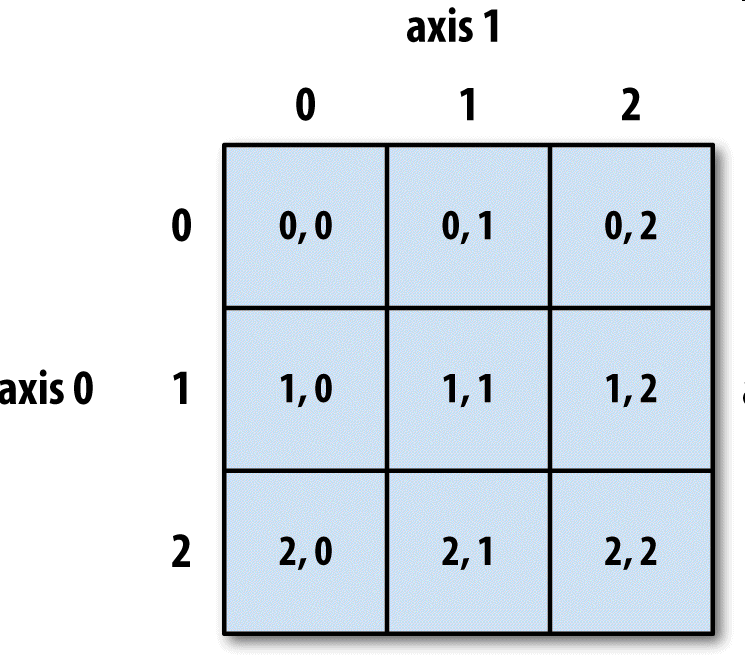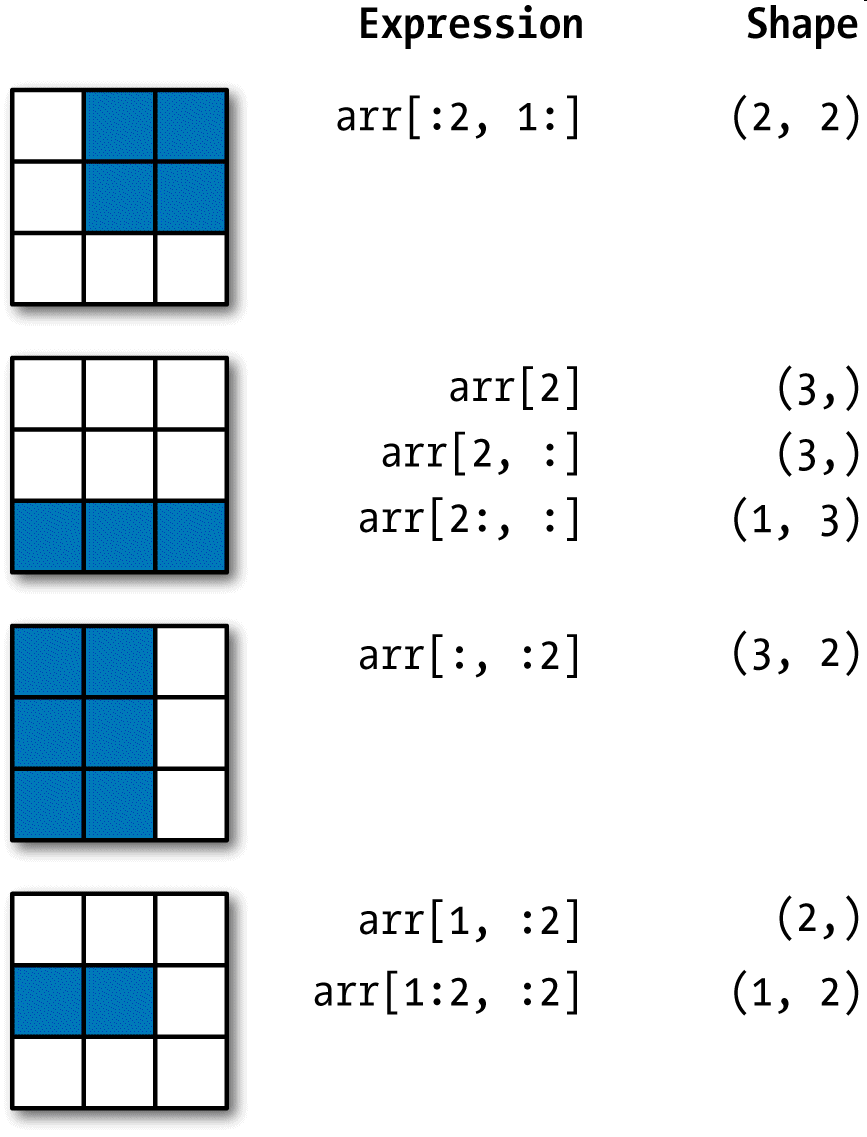﻿• 价格透明
• 信息保密
• 进度掌控
• 售后无忧# 【数据分析从入门到“入坑“系列】利用Python学习数据分析-Numpy中的索引

## 基本的索引和切片

NumPy数组的索引是一个内容丰富的主题，因为选取数据子集或单个元素的方式有很多。一维数组很简单。从表面上看，它们跟Python列表的功能差不多：

```In : arr = np.arange(10)
​
In : arr
Out: array([0, 1, 2, 3, 4, 5, 6, 7, 8, 9])
​
In : arr
Out: 5
​
In : arr[5:8]
Out: array([5, 6, 7])
​
In : arr[5:8] = 12
​
In : arr
Out: array([ 0,  1,  2,  3,  4, 12, 12, 12,  8,  9])```

```In : arr_slice = arr[5:8]
​
In : arr_slice
Out: array([12, 12, 12])```

```In : arr_slice = 12345
​
In : arr
Out: array([    0,     1,     2,     3,     4,    12, 12345,    12,     8,
9])```

```In : arr_slice[:] = 64
​
In : arr
Out: array([ 0,  1,  2,  3,  4, 64, 64, 64,  8,  9])```

```In : arr2d = np.array([[1, 2, 3], [4, 5, 6], [7, 8, 9]])
​
In : arr2d
Out: array([7, 8, 9])```

```In : arr2d
Out: 3
​
In : arr2d[0, 2]
Out: 3``````In : arr3d = np.array([[[1, 2, 3], [4, 5, 6]], [[7, 8, 9], [10, 11, 12]]])
​
In : arr3d
Out:
array([[[ 1,  2,  3],
[ 4,  5,  6]],
[[ 7,  8,  9],
[10, 11, 12]]])```

arr3d是一个2×3数组：

```In : arr3d
Out:
array([[1, 2, 3],
[4, 5, 6]])```

```In : old_values = arr3d.copy()
​
In : arr3d = 42
​
In : arr3d
Out:
array([[[42, 42, 42],
[42, 42, 42]],
[[ 7,  8,  9],
[10, 11, 12]]])
​
In : arr3d = old_values
​
In : arr3d
Out:
array([[[ 1,  2,  3],
[ 4,  5,  6]],
[[ 7,  8,  9],
[10, 11, 12]]])```

```In : arr3d[1, 0]
Out: array([7, 8, 9])```

```In : x = arr3d
​
In : x
Out:
array([[ 7,  8,  9],
[10, 11, 12]])
​
In : x
Out: array([7, 8, 9])```

## 切片索引

ndarray的切片语法跟Python列表这样的一维对象差不多：

```In : arr
Out: array([ 0,  1,  2,  3,  4, 64, 64, 64,  8,  9])
​
In : arr[1:6]
Out: array([ 1,  2,  3,  4, 64])```

```In : arr2d
Out:
array([[1, 2, 3],
[4, 5, 6],
[7, 8, 9]])
​
In : arr2d[:2]
Out:
array([[1, 2, 3],
[4, 5, 6]])```

```In : arr2d[:2, 1:]
Out:
array([[2, 3],
[5, 6]])```

```In : arr2d[1, :2]
Out: array([4, 5])```

```In : arr2d[:2, 2]
Out: array([3, 6])```

```In : arr2d[:, :1]
Out:
array([,
,
])``````In : arr2d[:2, 1:] = 0
​
In : arr2d
Out:
array([[1, 0, 0],
[4, 0, 0],
[7, 8, 9]])```

## 布尔型索引

```In : names = np.array(['Bob', 'Joe', 'Will', 'Bob', 'Will', 'Joe', 'Joe'])
​
In : data = np.random.randn(7, 4)
​
In : names
Out:
array(['Bob', 'Joe', 'Will', 'Bob', 'Will', 'Joe', 'Joe'],
dtype='<U4')
​
In : data
Out:
array([[ 0.0929,  0.2817,  0.769 ,  1.2464],
[ 1.0072, -1.2962,  0.275 ,  0.2289],
[ 1.3529,  0.8864, -2.0016, -0.3718],
[ 1.669 , -0.4386, -0.5397,  0.477 ],
[ 3.2489, -1.0212, -0.5771,  0.1241],
[ 0.3026,  0.5238,  0.0009,  1.3438],
[-0.7135, -0.8312, -2.3702, -1.8608]])```

```In : names == 'Bob'
Out: array([ True, False, False,  True, False, False, False], dtype=bool)```

```In : data[names == 'Bob']
Out:
array([[ 0.0929,  0.2817,  0.769 ,  1.2464],
[ 1.669 , -0.4386, -0.5397,  0.477 ]])```

```In : data[names == 'Bob']
Out:
array([[ 0.0929,  0.2817,  0.769 ,  1.2464],
[ 1.669 , -0.4386, -0.5397,  0.477 ]])```

```In : data[names == 'Bob', 2:]
Out:
array([[ 0.769 ,  1.2464],
[-0.5397,  0.477 ]])
​
In : data[names == 'Bob', 3]
Out: array([ 1.2464,  0.477 ])```

```In : names != 'Bob'
Out: array([False,  True,  True, False,  True,  True,  True], dtype=bool)
​
In : data[~(names == 'Bob')]
Out:
array([[ 1.0072, -1.2962,  0.275 ,  0.2289],
[ 1.3529,  0.8864, -2.0016, -0.3718],
[ 3.2489, -1.0212, -0.5771,  0.1241],
[ 0.3026,  0.5238,  0.0009,  1.3438],
[-0.7135, -0.8312, -2.3702, -1.8608]])```

~操作符用来反转条件很好用：

```In : cond = names == 'Bob'
​
In : data[~cond]
Out:
array([[ 1.0072, -1.2962,  0.275 ,  0.2289],
[ 1.3529,  0.8864, -2.0016, -0.3718],
[ 3.2489, -1.0212, -0.5771,  0.1241],
[ 0.3026,  0.5238,  0.0009,  1.3438],
[-0.7135, -0.8312, -2.3702, -1.8608]])```

```In : mask = (names == 'Bob') | (names == 'Will')
​
Out: array([ True, False,  True,  True,  True, False, False], dtype=bool)
​
Out:
array([[ 0.0929,  0.2817,  0.769 ,  1.2464],
[ 1.3529,  0.8864, -2.0016, -0.3718],
[ 1.669 , -0.4386, -0.5397,  0.477 ],
[ 3.2489, -1.0212, -0.5771,  0.1241]])```

```In : data[data < 0] = 0
​
In : data
Out:
array([[ 0.0929,  0.2817,  0.769 ,  1.2464],
[ 1.0072,  0.    ,  0.275 ,  0.2289],
[ 1.3529,  0.8864,  0.    ,  0.    ],
[ 1.669 ,  0.    ,  0.    ,  0.477 ],
[ 3.2489,  0.    ,  0.    ,  0.1241],
[ 0.3026,  0.5238,  0.0009,  1.3438],
[ 0.    ,  0.    ,  0.    ,  0.    ]])```

```In : data[names != 'Joe'] = 7
​
In : data
Out:
array([[ 7.    ,  7.    ,  7.    ,  7.    ],
[ 1.0072,  0.    ,  0.275 ,  0.2289],
[ 7.    ,  7.    ,  7.    ,  7.    ],
[ 7.    ,  7.    ,  7.    ,  7.    ],
[ 7.    ,  7.    ,  7.    ,  7.    ],
[ 0.3026,  0.5238,  0.0009,  1.3438],
[ 0.    ,  0.    ,  0.    ,  0.    ]])```

## 花式索引

```In : arr = np.empty((8, 4))
​
In : for i in range(8):
.....:     arr[i] = i
​
In : arr
Out:
array([[ 0.,  0.,  0.,  0.],
[ 1.,  1.,  1.,  1.],
[ 2.,  2.,  2.,  2.],
[ 3.,  3.,  3.,  3.],
[ 4.,  4.,  4.,  4.],
[ 5.,  5.,  5.,  5.],
[ 6.,  6.,  6.,  6.],
[ 7.,  7.,  7.,  7.]])```

```In : arr[[4, 3, 0, 6]]
Out:
array([[ 4.,  4.,  4.,  4.],
[ 3.,  3.,  3.,  3.],
[ 0.,  0.,  0.,  0.],
[ 6.,  6.,  6.,  6.]])```

```In : arr[[-3, -5, -7]]
Out:
array([[ 5.,  5.,  5.,  5.],
[ 3.,  3.,  3.,  3.],
[ 1.,  1.,  1.,  1.]])```

```In : arr = np.arange(32).reshape((8, 4))
​
In : arr
Out:
array([[ 0,  1,  2,  3],
[ 4,  5,  6,  7],
[ 8,  9, 10, 11],
[12, 13, 14, 15],
[16, 17, 18, 19],
[20, 21, 22, 23],
[24, 25, 26, 27],
[28, 29, 30, 31]])
​
In : arr[[1, 5, 7, 2], [0, 3, 1, 2]]
Out: array([ 4, 23, 29, 10])```

```In : arr[[1, 5, 7, 2]][:, [0, 3, 1, 2]]
Out:
array([[ 4,  7,  5,  6],
[20, 23, 21, 22],
[28, 31, 29, 30],
[ 8, 11,  9, 10]])```### 低价透明### 金牌服务### 信息保密